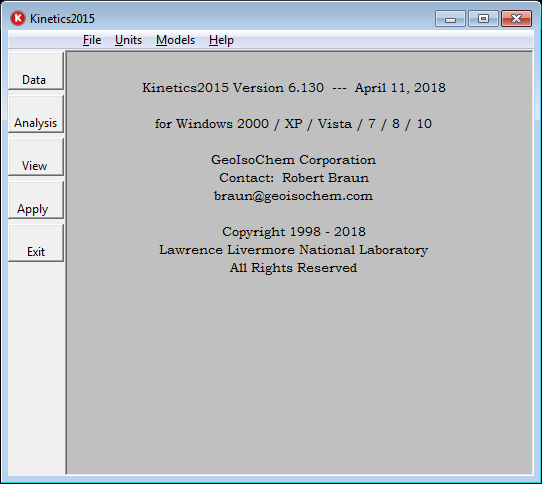# آنالیز کینتیکس | Kinetics 2015

نوشته شده توسط Super User. ارسال در مهندسی نفت و مخزنKinetics 2015 a Computer Program to Analyze Chemical Reaction Data, user-friendly kinetics analysis computer package designed for reactions such as kerogen, polymer, or mineral decomposition. It can fit rate parameters to chemical reaction data (rate or cumulative reacted) measured at a series of constant temperatures, constant heating rates, or arbitrary thermal histories. In addition to several approximate methods of kinetics analysis, six nonlinear regression methods are available.The nonlinear regression analysis methods range from a single first-order reaction to a variety of activation energy distribution models. The nth-order reaction model can fit activation energy, frequency factor, and reaction order (including n=1) for up to three parallel reactions.

The nth-order model is equivalent to a Gamma distribution model. The Gaussian activation energy distribution model can fit those three parameters plus a Gaussian distribution parameter for up to three parallel reactions. The Weibull distribution model fits a frequency factor, mean activation energy, a scale parameter related to width, and a distribution shape parameter for up to three parallel reactions. The Discrete-distribution model fits an average frequency factor and relative fractions and activation energies for up to 25 parallel, first-order reactions. One option of the Discrete model allows the frequency factor to increase exponentially with activation energy.

The Nucleation-Growth model fits a frequency factor, an activation energy, a nucleation parameter, and a reaction order for up to three parallel reactions. This model is particularly useful for the kinetics analysis of linear polymers, cellulose, and some well-preserved algal kerogens, which have a reaction-rate profile that is narrower than that for a single first-order reaction. A new variation of the Nucleation-Growth model for Kinetics2015 includes a thermodynamic inhibition term (1-1/K), which is particularly useful for deriving phase transition models. Finally, the

alternate-pathway model (A --> C, A --> B, and B --> C) can be used for a variety of purposes, including fitting narrow reaction-rate profiles and fitting serial reactions by constraining the A --> B frequency factor to zero.

When analyzing experiments conducted at a series of constant heating rates, the program uses a very fast approximate fitting procedure to determine possible initial parameter estimates for the subsequent nonlinear regression analysis. This increases the probability that the regression analysis will

Properly converge with a minimum of computer time. Once convergence is reached by the discrete model, the parameter space is further systematically searched to achieve global convergence. With the Gaussian, nth-order, Weibull, nucleation, and alternate-pathway models, the calculated rates or integrals can be convoluted with an experimental tracer signal during the nonlinear regression to account for dispersion effects often found in real chemical reaction data.

تیم PetroTools آخرین نسخه این نرم افزار را برای شما ارائه می دهد. جهت تهیه نرم افزار با استفاده از منوی خرید با ما در تماس باشید

### درباره PetroToolsتیم PetroTools با داشتن کادری مجرب، ارائه دهنده کلیه نرم افزارهای مهندسی نفت، مخزن، زمین شناسی، پتروفیزیک، لرزه نگاری و غیره می باشد. جهت تهیه نرم افزارهای مورد نیاز خود، از طریق منوی تماس با ما در خواست خود را ارسال کنید.